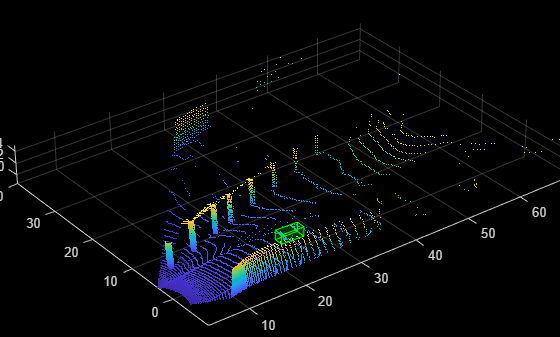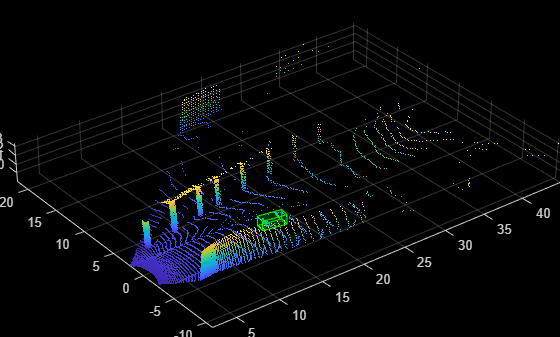# Data Augmentations for Lidar Object Detection Using Deep Learning

This example shows how to perform typical data augmentation techniques used in 3-D object detection workflows with lidar data.

Lidar object detection methods directly predict 3-D bounding boxes around the objects of interest. Data augmentation methods can help you improve prediction accuracy and avoid overfitting issues while training. This example covers global and local augmentation techniques: global augmentation techniques are applied to the entire point cloud of a scene and local augmentation techniques are applied only to points belonging to individual objects in the scene.

Extract the ZIP file attached to this example to the temp directory.

```unzip('sampleWPIPointClouds.zip',tempdir); dataLocation = fullfile(tempdir,'sampleWPIPointClouds');```

Create a file datastore to load PCD files using the `pcread` function.

`lds = fileDatastore(dataLocation,'ReadFcn',@(x) pcread(x));`

Create a box label datastore for loading the 3-D ground truth bounding boxes.

```load('sampleWPILabels.mat','trainLabels') bds = boxLabelDatastore(trainLabels);```

Use the `combine` function to combine the point clouds and 3-D bounding boxes into a single datastore.

`cds = combine(lds,bds);`

Display the point cloud.

```inputData = read(cds); ptCloud = inputData{1,1}; gtLabels = inputData{1,2}; figure; ax = pcshow(ptCloud.Location);```

Draw the 3-D bounding boxes over the point cloud.

```showShape('cuboid',gtLabels,'Parent',ax,'Opacity',0.1, ... 'Color','green','LineWidth',0.5); zoom(ax,2);````reset(cds);`

### Global Data Augmentation

Global data augmentation techniques are used when the point clouds in a dataset have little variation. A global technique applies a transformation to the entire point cloud to generate new samples of the point cloud that are not present in the original data set. The same transformation is applied to all corresponding ground truth boxes. The following four global data augmentation techniques are commonly used .

1. Random rotation

2. Random scaling

3. Random translation

4. Random flipping

#### Random Rotation of Point Cloud

Randomly rotate the point cloud and the 3-D bounding boxes within the specified range of angles along the z-axis. By randomly rotating the point cloud, you can simulate data points, such as a vehicle taking a turn. The typical range for rotation is `[-45 45]` degrees.

Set the random seed for reproducibility.

`rng(1);`

Define minimum and maximum yaw angles for rotation.

```minYawAngle = -45; maxYawAngle = 45;```

Define the grid size to bin the point cloud to.

`gridSize = [32 32 32];`

Define the limits of the region of interest within the point cloud.

`axisLimits = [-100 100];`

Create an output view for the affine transformation.

`outView = imref3d(gridSize,axisLimits,axisLimits,axisLimits);`

Calculate a random angle from the specified yaw angle range.

`theta = minYawAngle + rand*(maxYawAngle - minYawAngle)`
```theta = -7.4680 ```

Create a transformation that rotates the point clouds and 3-D bounding boxes.

`tform = randomAffine3d('Rotation',@() deal([0,0,1],theta));`

Apply the transformation to the point cloud.

`ptCloudTransformed = pctransform(ptCloud,tform);`

Apply the same transformation to the 3-D bounding boxes.

`gtLabelsTranformed = bboxwarp(gtLabels,tform,outView); `

Display the rotated point cloud and the ground truth boxes.

```figure; ax1 = pcshow(ptCloudTransformed.Location); showShape('cuboid',gtLabelsTranformed,'Parent',ax1,'Opacity',0.1, ... 'Color','green','LineWidth',0.5); zoom(ax1,2);```#### Random Scaling of Point Cloud

Randomly scale the point cloud and the 3-D bounding boxes from the specified range of scales. The typical scaling range is `[0.95 1.05]`. The example uses the range `[0.5 0.7]` for better visualization.

Create a transformation to scale the point cloud and 3-D bounding boxes.

`tform = randomAffine3d('Scale',[0.5 0.7]);`

Apply the transformation to the point cloud.

`ptCloudTransformed = pctransform(ptCloud,tform);`

Apply the same transformation to the 3-D bounding boxes.

`gtLabelsTranformed = bboxwarp(gtLabels,tform,outView); `

Display the scaled point cloud and the ground truth boxes.

```figure; ax2 = pcshow(ptCloudTransformed.Location); showShape('cuboid',gtLabelsTranformed,'Parent',ax2,'Opacity',0.1, ... 'Color','green','LineWidth',0.5); zoom(ax2,2);```#### Random Translation of Point Cloud

Randomly translate the point cloud and the 3-D bounding boxes along the x-, y-, and z-axis from the specified range.

Create a transformation to translate the point cloud and 3-D bounding boxes.

```tform = randomAffine3d('XTranslation',[0 0.2],... 'YTranslation',[0 0.2],... 'ZTranslation',[0 0.1]); ```

Apply the transformation to the point cloud.

`ptCloudTransformed = pctransform(ptCloud,tform);`

Apply the same transformation to the 3-D bounding boxes.

`gtLabelsTranformed = bboxwarp(gtLabels,tform,outView); `

Display the translated point cloud and the ground truth boxes.

```figure; ax3 = pcshow(ptCloudTransformed.Location); showShape('cuboid',gtLabelsTranformed,'Parent',ax3,'Opacity',0.1,'Color', ... 'green','LineWidth',0.5); zoom(ax3,2);```#### Random Flipping Along Axis

Randomly flip the point cloud and the 3-D bounding boxes along the y-axis. Do not flip along the x-axis, as the bounding box annotations are provided in the camera field of view.

Create a transformation to flip the point cloud and 3-D bounding boxes.

`tform = randomAffine3d('YReflection',true);`

Apply the transformation to the point cloud.

`ptCloudTransformed = pctransform(ptCloud,tform);`

Apply the same transformation to the 3-D bounding boxes using the helper function `flipBbox`, attached to this example as a supporting file.

`gtLabels = flipBbox(gtLabels,tform);`

Display the flipped point cloud and the ground truth boxes.

```figure; ax4 = pcshow(ptCloudTransformed.Location); showShape('cuboid',gtLabels,'Parent',ax4,'Opacity',0.1, ... 'Color','green','LineWidth',0.5); zoom(ax4,2);```### Ground Truth Data Augmentation

Ground truth data augmentation is a technique where during training, randomly selected ground truth boxes from other point clouds are introduced into the current training point cloud . Using this approach, you can increase the number of ground truth boxes per point cloud and simulate objects existing in different environments. To avoid physically impossible outcomes, you perform a collision test on the samples to be added and the ground truth boxes of the current point cloud. Use this augmentation technique when there is a class imbalance in the data set.

Use the `generateGTDataForAugmentation` and `groundTruthDataAugmentation` helper functions, attached to this example as supporting files, to randomly add a fixed number of objects to the point cloud from the car class.

```trainData = lds.readall; gtData = generateGTDataForAugmentation(trainData,trainLabels);```

Define the number of ground truth boxes to add.

```numSamplesToAdd = 3; cdsAugmented = transform(cds,@(x) groundTruthDataAugmenation(x,gtData,numSamplesToAdd));```

Display the point cloud along with the ground truth augmented boxes.

```augData = read(cdsAugmented); augptCld = augData{1,1}; augLabels = augData{1,2}; figure; ax5 = pcshow(augptCld(:,1:3)); showShape('cuboid',augLabels,'Parent',ax5,'Opacity',0.1, ... 'Color','green','LineWidth',0.5); zoom(ax5,2);```### Local Data Augmentation

Local data augmentation applies augmentation only to the points inside the ground truth boxes, not to the entire point cloud . Local data augmentation can be used when you want to apply the transformations only to the points inside the ground truth boxes. The rest of the point cloud remains the same.

Read the point cloud and corresponding ground truth label.

```inputData = read(cds); ptCloud = inputData{1,1}; gtLabels = inputData{1,2}; gtLabelsTransformed = zeros(size(gtLabels)); for i = 1:size(gtLabels,1) labelParams = gtLabels(i,:); centroidLoc = labelParams(1,1:3); model = cuboidModel(labelParams); indices = findPointsInsideCuboid(model, ptCloud); numPointsInside = size(indices,1); ```

Segregate the ground truth points from the original point cloud using the helper funtion `removeIndicesFromPointCloud`, attached to this example as a supporting file.

``` updatedPtCloud = removeIndicesFromPtCloud(ptCloud,indices); cubPtCloud = select(ptCloud,indices); % Shift the segregrated point cloud to the origin. numPoints = cubPtCloud.Count; shiftRange = -1.*repmat(centroidLoc,[numPoints 1]); cubPtCloud = pctransform(cubPtCloud,shiftRange); % Define the minimum and maximum yaw angles for rotation. minYawAngle = -45; maxYawAngle = 45; % Calculate a random angle from the specified yaw angle range. theta = minYawAngle + rand*(maxYawAngle - minYawAngle); % Create a transformation that rotates, translates, and scales the % point clouds and 3-D bounding boxes. tform = randomAffine3d('Rotation',@() deal([0,0,1],theta),... 'Scale',[0.95,1.05],... 'XTranslation',[0,0.2],... 'YTranslation',[0,0.2],... 'ZTranslation',[0,0.1]); % Apply the transfomation to the 3-D bounding box. labelParams(1,1:3) = labelParams(1,1:3) - centroidLoc; labelParamsTransformed = bboxwarp(labelParams,tform,outView); % Calculate the overlap ratio between the transformed box and the % original ground truth boxes by converting them to rotated rectangle % format, defined as [xcenter,ycenter,width,height,yaw]. overlapRatio = bboxOverlapRatio(labelParamsTransformed(:,[1,2,4,5,9]), ... gtLabels(:,[1,2,4,5,9])); [maxOverlapRatio, maxOverlapIdx] = max(overlapRatio); % Check to see if any transformed boxes overlap with the ground truth % boxes. if (maxOverlapRatio > 0) && (maxOverlapIdx ~= i) shiftRange = -1.*shiftRange; cubPtCloud = pctransform(cubPtCloud,shiftRange); updatedPtCloud = pccat([updatedPtCloud,cubPtCloud]); gtLabelsTransformed(i,1) = labelParams; else cubPtCloudTransformed = pctransform(cubPtCloud,tform); shiftRange = -1.*shiftRange; cubPtCloudTransformed = pctransform(cubPtCloudTransformed,shiftRange); updatedPtCloud = pccat([updatedPtCloud,cubPtCloudTransformed]); gtLabelsTransformed(i,:) = labelParamsTransformed; end gtLabelsTransformed(i,1:3) = gtLabelsTransformed(i,1:3) + centroidLoc; ptCloud = updatedPtCloud; end```

Display the point cloud along with the augmented ground truth boxes.

```figure; ax6 = pcshow(updatedPtCloud.Location); showShape('cuboid',gtLabelsTransformed,'Parent',ax6,'Opacity',0.1, ... 'Color','green','LineWidth',0.5); zoom(ax6,2);````reset(cds);`

### References

 Hahner, Martin, Dengxin Dai, Alexander Liniger, and Luc Van Gool. "Quantifying Data Augmentation for LiDAR Based 3D Object Detection." Preprint, submitted April 3, 2020. https://arxiv.org/abs/2004.01643.

## SupportGet trial now